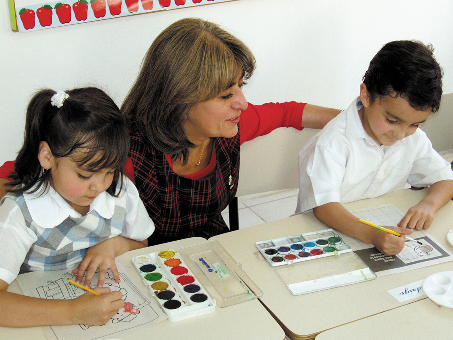,
Message sent from:Maths Key Stage 3 Aims Pupils in KS3 will be following the New Maths National Curriculum for 2014 using the Edexcel Maths Progress Scheme Of Work and resources. The resources are split into three pathways: Pi, Theta and Delta with Alpha supporting the lower ability students. The emphasis in this programme of study is to prepare pupils for KS4 and to develop numeracy skills. Because there is a requirement in the new National Curriculum for pupils to reasoning mathematically , certain topics will be taught using the bar modelling method from the Singapore Maths Programme of study. This will allow pupils to use a concrete to pictorial to abstract approach that gives them a sound basis for problem solving in KS4. Year 7 Theta 1(For pupils at L4 at end of KS2) Autumn Term 1 – Unit 1: Analysing and Displaying Data Unit 2: Number Skills Autumn Term 2 – Unit 3: Expressions, functions and formulae Unit 4: Decimals and measures. Spring Term 1 – Unit 5 Fractions Unit 6 Probability Spring Term 2 – Unit 7 Ratio and proportion Unit 8 Lines and angles Summer Term 1 – Unit 9 Sequences and graphs Unit 10 Transformations Summer Term 2 – Unit 1 (Theta 2) Number Unit 2 (Theta 2) Area and Volume Year 8 Theta 2 Autumn Term 1 – Unit 6 Decimals and ratio Unit 4 Expressions and equations. Autumn Term 2 – Unit 3 Statistics, graphs and charts Unit 5 Real-life graphs Spring Term 1 – Unit 7 Lines and angles Unit 8 Calculating with fractions Spring Term 2 – Unit 9 Straight-line graphs Unit 10 Percentages, decimals and fractions Summer Term 1 – Unit 1(Theta 3) Indices and standard form Unit 2 (Theta 3)Expressions and formulae Summer Term 2 – Unit 3(Theta 3) Dealing with data Unit 4(Theta 3) Multiplicative reasoning Year 9 Theta 3 Autumn Term 1 – Unit 5 Constructions Unit 6 Equations, inequalities and proportionality Autumn Term 2 – Unit 7 Circles, Pythagoras and prisms Unit 8 Sequences and graphs Spring Term 1 – Unit 9 Probability Unit 10 Comparing shapes Spring Term 2 – Unit 1(Delta 1)Analysing and Displaying Data Unit 2(Delta 1) Number skills Summer Term 1 – Unit 4(Delta 1)Fractions Unit 6(Delta 1)decimals Summer Term 2 – Unit 8(Delta 1)Multiplicative Reasoning Unit 9(Delta 1) Perimeter, Area and Volume.